# High School Physics : Understanding Electric Fields

## Example Questions

### Example Question #1 : Understanding Electric Fields

Calculate the magnitude of the electric field at a point that is located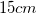directly north of a point charge,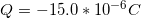.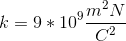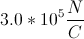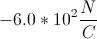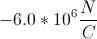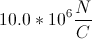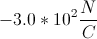Explanation:

The magnitude of an electric field due to a single point charge is given by the formula: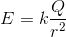The variablerefers to Coulomb's constant. We are given the charge and the distance between points. To use the equation, first convert the distance to meters.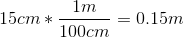We can now use the given constant, charge, and distance in the equation to solve for the magnetic field strength.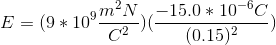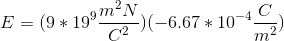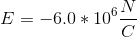### All High School Physics Resources